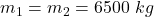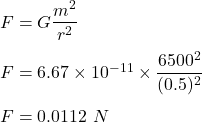## Q1) Calculate the gravitational force between two large trucks, each with a mass of 6500 kg, if they are parked next to each other sep

Question

Q1) Calculate the gravitational force between two large trucks, each with a mass of 6500 kg, if
they are parked next to each other separated by a distance of 0.5 m Ignore all other
gravitational forces.

in progress 0
6 months 2021-08-25T17:50:48+00:00 1 Answers 0 views 0

F = 0.0112 N

Explanation:

Given that,

Masses,The distance between masses, d = 0.5 m

We need to find the gravitational force between two large trucks. The formula for the gravitational forces is given by :So, the required force between masses is equal to 0.0112 N.